TitleCollege Algebra
Answer/Discussion to Practice Problems
Tutorial 54C: Arithmetic Sequences and
SeriesAnswer/Discussion to 1a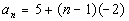Since an arithmetic sequence is a sequence, you find the terms exactly the same way that you do a sequence.   n is our term number and we plug the term number into the function to find the value of the term. If you need a review on sequences, feel free to go to Tutorial 54A: Sequences. Lets see what we get for our first five terms: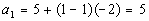*n = 1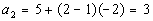*n = 2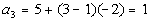*n = 3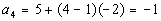*n = 4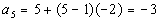*n = 5

 Note how the first term is 5. What was the common difference for this arithmetic sequence? If you said -2 you are correct!  Note how each term went DOWN by 2 from the previous term. Now lets check out the 15th term: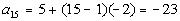*n = 15Answer/Discussion to 1b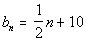Since an arithmetic sequence is a sequence, you find the terms exactly the same way that you do a sequence.   n is our term number and we plug the term number into the function to find the value of the term. If you need a review on sequences, feel free to go to Tutorial 54A: Sequences. Lets see what we get for our first five terms: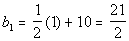*n = 1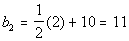*n = 2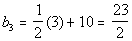*n = 3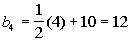*n = 4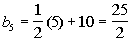*n = 5

 Note how the first term is 21/2. What was the common difference for this arithmetic sequence? If you said 1/2 you are correct!  Note how each term went up by 1/2 from the previous term. Now lets check out the 15th term: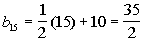*n = 15Answer/Discussion to 2a 0, .25, .5, .75, ...

 We will use the nth term formula for an arithmetic sequence,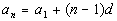, to help us with this problem. Basically we need to find two things, the first term of the sequence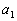and the common difference, d. What is, the first term of the sequence? If you said 0, give yourself a high five.  The first term of this sequence is 0. What is d, the common difference? If you said .25, you are right!!  Note that you would have to add .25 to each previous term to get to the next term: 0 + .25 = .25, .25 + .25 = .5, and .5 + .25 = .75.   It has to be consistent throughout the sequence. Putting in 0 forand .25 for d we get: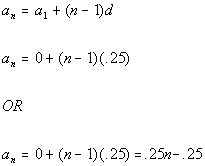Either of these answers would give you the same arithmetic sequence.Answer/Discussion to 2b -5, -15, -25, -35, ...

 We will use the nth term formula for an arithmetic sequence,, to help us with this problem. Basically we need to find two things, the first term of the sequenceand the common difference, d. What is, the first term of the sequence? If you said -5, give yourself a high five.  The first term of this sequence is -5. What is d, the common difference? If you said -10, you are right!!  Note that you would have to add -10 to each previous term to get to the next term: -5 + (-10) = -15, -15 + (-10) = -25, and -25 + (-10) = -35.   It has to be consistent throughout the sequence. Putting in -5 forand -10 for d we get: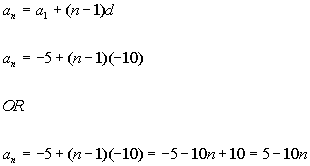Either of these answers would give you the same arithmetic sequence.Answer/Discussion to 3a 5 + 10 + 15 +.... + 500

 We will use the formula for the sum of the first n terms of an arithmetic sequence,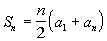, to help us with this problem. Basically we need to find three things: the first term of the sequence, the last term of the sequence, and how many terms of the sequence we are adding in the series. What is the first term? If you said 5 you are right!  What is the last term? If you said 500 give yourself a pat on the back. How many terms are we summing up? If you said 100, you are correct. Because of the way it is written with the 3 dots, this one is a little bit trickier.  You have to do a little figuring.  We can use the nth term of an arithmetic sequence and solve for n, the number of terms in the sequence: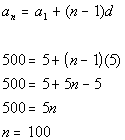Putting in 5 for the first term, 500 for the last term, and 100 for n, we get: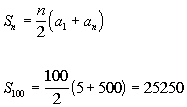Answer/Discussion to 3b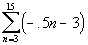Note how n starts at 3 and ends at 15.  So we are looking for the sum of terms 3 - 15. We will use the formula for the sum of the first n terms of an arithmetic sequence,, to help us with this problem. Basically we need to find three things: the first term of the sequence, the last term of the sequence, and how many terms of the sequence we are adding in the series. What is the first term? If you said -4.5 you are right!  Since this summation starts at 3, you need to plug in 3 into the given formula: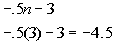What is the last term? If you said -10.5 give yourself a pat on the back. You get that by plugging 15 into the given formula: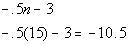How many terms are we summing up? If you said 13, you are correct.  If you start at 3 and go all the way to 15, there will be 13 terms. Putting in -4.5 for the first term, -10.5 for the last term, and 13 for n, we get: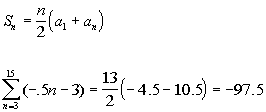Last revised on May 16, 2011 by Kim Seward.
All contents copyright (C) 2002 - 2011, WTAMU and Kim Seward. All rights reserved.